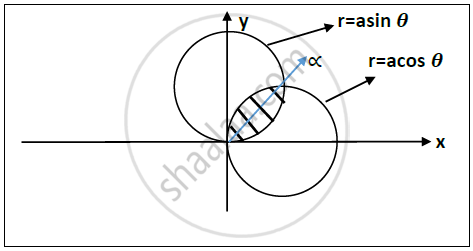Advertisement Remove all ads

# Use Polar Co Ordinates to Evaluate ∫ ∫ ( X 2 + Y 2 ) 2 X 2 Y 2 𝒅𝒙 𝒅𝒚 Over Yhe Area Common to Circle X 2 + Y 2 = a X and X 2 + Y 2 = B Y , a > B > 0 - Applied Mathematics 2

Sum

Use polar co ordinates to evaluate int int (x^2+y^2)^2/(x^2y^2) 𝒅𝒙 𝒅𝒚 over yhe area Common to circle x^2+y^2=ax  "and" x^2+y^2=by, a>b>0

Advertisement Remove all ads

#### Solution

Let I = int int (x^2+y^2)^2/(x^2y^2) 𝒅𝒙 𝒅𝒚
Region of integration is : Area common to the circle
x^2+y^2=ax  "and" x^2+y^2=by

To change the Cartesian coordinates to polar coordinates
Put x= r cos𝜽 and y = r sin 𝜽
Circles : r=acos 𝜽 𝒂𝒏𝒅 r=asin 𝜽
The function becomes : f(x,y) = (x^2+y^2)^2/(x^2y^2)=r^4/(r^4sin^2thetacos^2theta)=4/(sin^2 2theta)=f(r,theta)Intersection of both circles is at angle = tan^(-1)  a/b

Divide the region into two equal halves.

For one region ,
𝟎≤𝒓≤𝒃𝒔𝒊𝒏𝜽
𝟎≤𝜽≤𝜶
For another region ,
𝟎≤𝒓≤𝒂𝒄𝒐𝒔 𝜽
𝜶≤𝜽≤pi/2

therefore "I"=int_0^\alphaint_0^(bsintheta)(4rdrd theta)/(sin^2 2theta) + int_0^(acostheta) int_alpha^(pi/2)(4rdrd theta)/(sin^2 2theta)

therefore "I"=int_0^alpha 4/(sin^2 2theta)[r^2/2]_0^(bsin theta)d theta+int_0^(pi/2)4/(sin^2 2theta)[r^2/2]_0^(acostheta)d theta

=1/2b^2int_0^alphasec^2theta d theta+a^2/2int_alpha^(pi/2)cosec^2theta d theta

=1/2b^2tanalpha+a^2/2cotalpha

=(ab)/2+(ab)/2`

∴ I = ab

Concept: Application of Double Integrals to Compute Area
Is there an error in this question or solution?
Advertisement Remove all ads

#### APPEARS IN

Advertisement Remove all ads
Advertisement Remove all ads
Share
Notifications

View all notifications

Forgot password?